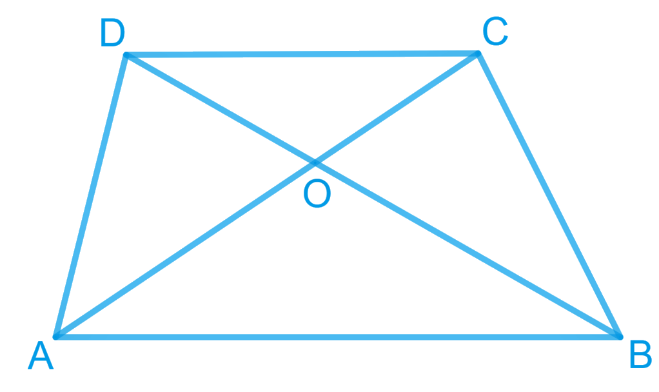# Ex.9.3 Q10 Areas of Parallelograms and Triangles Solution - NCERT Maths Class 9

## Question

Diagonals $$AC$$ and $$BD$$ of a trapezium $$ABCD$$ with $$AB\; || \;DC$$ intersect each other at $$O$$. Prove that $$ar (AOD) = ar (BOC)$$.

Video Solution
Areas Of Parallelograms And Triangles
Ex 9.3 | Question 10

## Text Solution

What is known?

Diagonals $$AC$$ and $$BD$$ of a trapezium $$ABCD$$ with $$AB || DC$$ intersect each other at $$O$$.

What is unknown?

How we can prove that $$ar (AOD) = ar (BOC).$$

Reasoning:

We can use theorem for triangles $$DAC$$ and $$DBC$$, if two triangles are on same base and between same pair of parallel lines then both will have equal area. Now we can subtract common area of triangle $$DOC$$ from both sides to get the required result.

Steps:It can be observed that \begin{align} (\Delta DAC)\, \rm{and} \,(\Delta DBC)\end{align} lie on the same base $$DC$$ and between the same parallels $$AB$$ and $$CD$$.

According to Theorem 9.2: Two triangles on the same base (or equal bases) and between the same parallels are equal in area.

\begin{align} {ar}\left( \Delta { DAC} \right)&={ar}\left( \Delta { DBC} \right) \\\left[ \begin{array} {ar}\left( \Delta { DAC} \right)- \\ {ar }\left( \Delta { DOC} \right) \\\end{array} \right]&\!\!=\!\!\left[ \begin{array} {ar }\left( \Delta { DBC} \right)- \\ {ar }\left( \Delta { DOC} \right) \\\end{array} \right] \\\end{align}

Subtracting area $$(\Delta{ DOC})$$ from both sides

\begin{align} {ar }\left( \Delta{ AOD} \right)&={ ar }\left( \Delta{ BOC} \right) \\\end{align}

Video Solution
Areas Of Parallelograms And Triangles
Ex 9.3 | Question 10
Learn from the best math teachers and top your exams

• Live one on one classroom and doubt clearing
• Practice worksheets in and after class for conceptual clarity
• Personalized curriculum to keep up with school